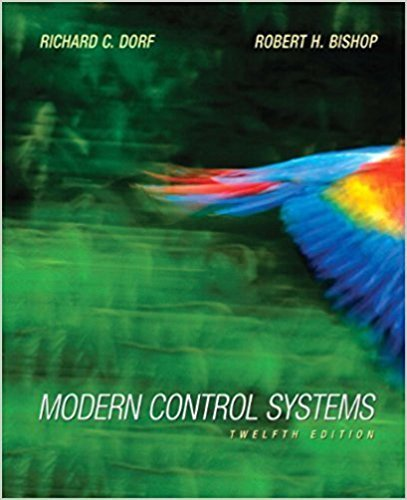×
×

# The speed control system of Figure DP4.1 is altered so that G(s) = l/(.v + 5) and theISBN: 9780136024583 453

## Solution for problem DP4.3 Chapter Chapter 4

Modern Control Systems | 12th Edition

• Textbook Solutions
• 2901 Step-by-step solutions solved by professors and subject experts
• Get 24/7 help from StudySoup virtual teaching assistantsModern Control Systems | 12th Edition

4 5 1 260 Reviews
31
4
Problem DP4.3

The speed control system of Figure DP4.1 is altered so that G(s) = l/(.v + 5) and the feedback is Ku as shown in Figure DP4.3. (a) Determine the range of /(| allowable so that the steady state is ess ^ 1 %. (b) Determine a suitable value for K^ and K so that the magnitude of the steady-state error to a wind disturbance Td{i) - 2t mrad/s, 0 < t < 5 s, is less than 0.1 mrad.

Step-by-Step Solution:
Step 1 of 3

“Circuit Analysis: Series and Parallel” Presented to The Faculty of the College of ECST California State University, Los Angeles In Partial Fulfillment of the Requirements...

Step 2 of 3

Step 3 of 3

##### ISBN: 9780136024583

Since the solution to DP4.3 from Chapter 4 chapter was answered, more than 261 students have viewed the full step-by-step answer. This full solution covers the following key subjects: . This expansive textbook survival guide covers 14 chapters, and 1211 solutions. This textbook survival guide was created for the textbook: Modern Control Systems, edition: 12. The full step-by-step solution to problem: DP4.3 from chapter: Chapter 4 was answered by , our top Engineering and Tech solution expert on 03/16/18, 03:13PM. Modern Control Systems was written by and is associated to the ISBN: 9780136024583. The answer to “The speed control system of Figure DP4.1 is altered so that G(s) = l/(.v + 5) and the feedback is Ku as shown in Figure DP4.3. (a) Determine the range of /(| allowable so that the steady state is ess ^ 1 %. (b) Determine a suitable value for K^ and K so that the magnitude of the steady-state error to a wind disturbance Td{i) - 2t mrad/s, 0 < t < 5 s, is less than 0.1 mrad.” is broken down into a number of easy to follow steps, and 79 words.

Unlock Textbook Solution